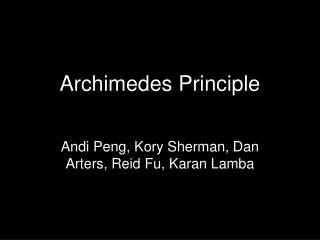DownloadDownload PresentationArchimedes Principle

# Archimedes Principle

Download Presentation## Archimedes Principle

- - - - - - - - - - - - - - - - - - - - - - - - - - - E N D - - - - - - - - - - - - - - - - - - - - - - - - - - -
##### Presentation Transcript

1. Archimedes Principle Andi Peng, Kory Sherman, Dan Arters, Reid Fu, Karan Lamba

2. In the third century BC, a Greek mathematician named Archimedes was put in charge of discovering if a goldsmith was secretly replacing the gold in the king’s crown with silver. Archimedes, in the middle of taking a bath, found that when he put his foot in the bathtub, some water rose and poured out of the tub. With this, he placed the king’s crown in water with a gold crown of equal weight. The king’s crown caused more water to overflow, and Archimedes therefore concluded that since it had more volume for the same weight, (gold is more dense than silver), the king’s crown contained silver. The goldsmith was executed.

3. Buoyancy-the upward force that keeps things afloat Archimedes law is the primary law of buoyancy The weight of the displaced fluid is directly proportional to the volume of the displaced fluid. Among submerged objects with equal mass, the objects with the greater volume will have greater buoyancy

4. Works Cited • http://en.wikipedia.org/wiki/Buoyancy • http://library.thinkquest.org/27948/archimede.html • http://library.thinkquest.org/25672/archi med.htm • http://www.mat.uc.pt/sabados/Imagens/Archimedes_principle.gif

5. Bernoulli’s Law By Jenn Furlong Grant Behnke Kody Keckler Victoria Ma Shyam Kotak

6. What is Bernoulli’s Law? As a fluid’s velocity increases, the pressure it exerts decreases (and vice versa). A common application is a Venturi tube, which narrows at one point; the pressure decreases, and velocity increases.

7. When does it apply? • The construction of airplane wings and race cars use this law by controlling pressure on the airplane wings and wheels. • This law applies when fluid passes through a gap in the pathway—pressure increases and decreases depending on the velocity of the fluid.

8. The Equation P1 + ½ pv12 + pgh1 = P2 + ½ pv 22 + pgh 2

9. Who was Bernoulli? • Daniel Bernoulli was born in Groningen, in the Netherlands. • From a young age, he was interested in mathematics and fluids. • He discovered the fluid equation when he went back to his earlier work on the Conservation of energy, and realized that a moving fluid exchanges kinetic energy for pressure similarly with how a fluid would exchange kinetic energy in a height gain.

10. Works Cited • http://hyperphysics.phy-astr.gsu.edu/HBASE/pber.html • http://en.wikipedia.org/wiki/Bernoulli's_equation • http://library.thinkquest.org/27948/bernoulli.html • http://pilotsweb.com/principle/bernoulli.htm • http://hyperphysics.phy-astr.gsu.edu/HBASE/pber.html • http://pass.maths.org.uk/issue1/bern/index.html

11. Pascal's Law • States that if pressure is applied to any part of a contained fluid, that pressure is applied to all parts of the fluid • P1=P2, P1 being the pressure at the point it’s applied at, P2 being the pressure at any other point of the enclosed fluid • An application of this is the hydraulic lift, when using Pascal’s Law, you can increase the area on one side, you could lift many times the weight with only a fraction of the effort

12. A French mathematician and Philosopher • He did work in many areas of math and science • Was a defender of Jansenism • Had poor health despite his accomplishments and died at age 39 • Contributed to some inventions including the barometer and mechanical calculator Blaise Pascal

13. Works Cited • Nave, R. "Pressure." Test Page for Apache Installation. 27 Mar. 2009 <http://hyperphysics.phy-astr.gsu.edu/hbase/pasc.html>. • "Cycloid -." Wikipedia, the free encyclopedia. 27 Mar. 2009 <http://en.wikipedia.org/wiki/Cycloid>. • "Blaise Pascal -." Wikipedia, the free encyclopedia. 27 Mar. 2009 <http://en.wikipedia.org/wiki/Blaise_Pascal>. • "Pascal's Principle and Hydraulics." NASA - Title... 27 Mar. 2009 <http://www.grc.nasa.gov/WWW/K-12/WindTunnel/Activities/Pascals_principle.html>. • Educational Technology Clearinghouse. 27 Mar. 2009 <http://etc.usf.edu/clipart/36100/36164/pascals_law_36164_lg.gif>. • "Pascal's law -." Wikipedia, the free encyclopedia. 27 Mar. 2009 <http://en.wikipedia.org/wiki/Pascal's_law>.

14. Robert Boyle’s Law that has to do with gases called Boyle’s Law(Ryan Friedman came up with the name) Ryan Carson Liz Eder Ryan Friedman Sivan Mills

15. What is Boyle’s Law? • Gases have physical properties that are related to each other. • Boyle’s Law says that when a gas’ mass and temperature stays the same, pressure and volume are proportionally inverse. • As pressure increases, volume decreases and vice versa.

16. Equation and Animation • P=Pressure • V=Volume • P1xV1=P2xV2

17. Work Citied • http://www.grc.nasa.gov/WWW/K-12/airplane/aboyle.html • http://en.wikipedia.org/wiki/Boyle's_law

18. Charles Law in Charge Law discovered by: Jacques-Alexandre-Cesar Charles

19. What does it do? At a constant pressure the volume of a gas is directly proportional to its temperature. V/ T=k As temperature increases volume increases. Charles came up with the idea to test it when he was in a hot air balloon which is a use of the law.

20. Sources • http://chemed.chem.purdue.edu/genchem/topicreview/bp/ch4/gaslaws3.html#charles